### Special Relativity

Now that we have unlocked the Lorentz transformation and discovered the frame effects of length contraction and time dilation, let's get back to our laser-taggers: Alyssa, Bev, and Crater.

They're done practicing, and today is game day. They're about to start a $3$-on-$3$ battle round, in which they have to knock out two out of three of their competitors to win the round. They score a KO when an opponent is hit simultaneously with two laser bolts.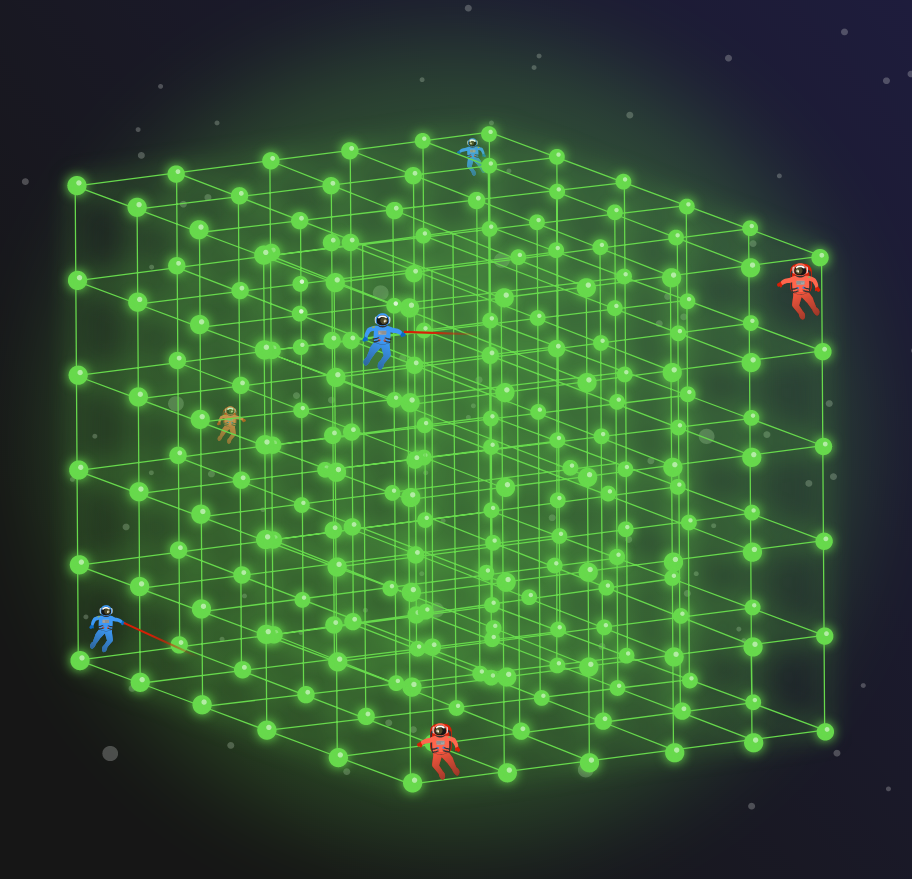The standard issue jetpack for relativistic laser tag has two speeds: $0$ and $\frac c2$ (relative to the reference frame of the arena). Players must stay within a $5$ light-second cubed volume $($measured when jetpack is set to $0$ speed$).$

Our team can only defeat their opponents using the relativistic tools we've developed in this chapter.

# Relativistic Laser Tag

During the game, flying out of bounds results in severe penalties. Before the competition, players have a chance to position themselves throughout the competition area, and get a feel for the size of the space. In a particular frame, the sides of the space have length $5$ light-seconds.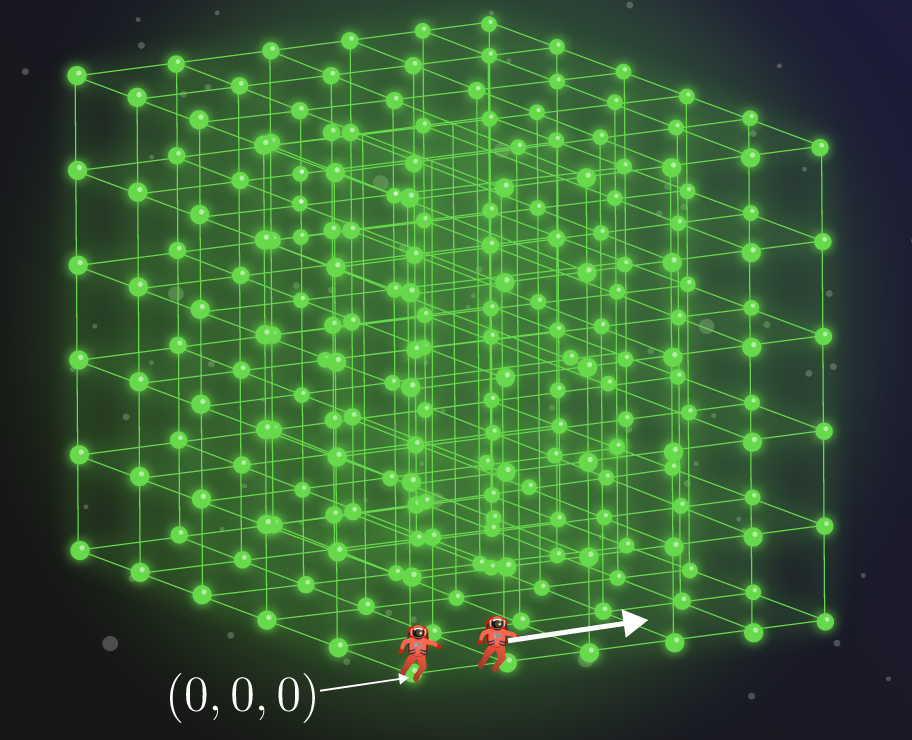Bev is at position $(0,0,0)$ and at rest relative to the playing space. She kicks on her jetpack to speed $\frac c2$ and starts off in the $x$-direction. In her reference frame, how far (in light-seconds) can she continue in this direction before she goes out of bounds?

# Relativistic Laser Tag

A light-second is a unit of distance equal to how far light travels in $\SI{1}{\second}.$

Alyssa remains at $(0,0,0)$ watching Bev traverse the entire arena at speed $\frac c2.$ For how long (in $\si{\second}$ according to Alyssa) is Bev moving until she stops at $(5,0,0)?$

Assume the time for Bev to accelerate and decelerate is negligible.

# Relativistic Laser Tag

This is not the amount of time that ticks by on the clock carried by Bev. How much time has passed on Bev's clock after she arrives on the other side of the arena, near point $(5,0,0)?$

Details: Bev's speed as she traversed the arena was $V=\frac c2.$

# Relativistic Laser Tag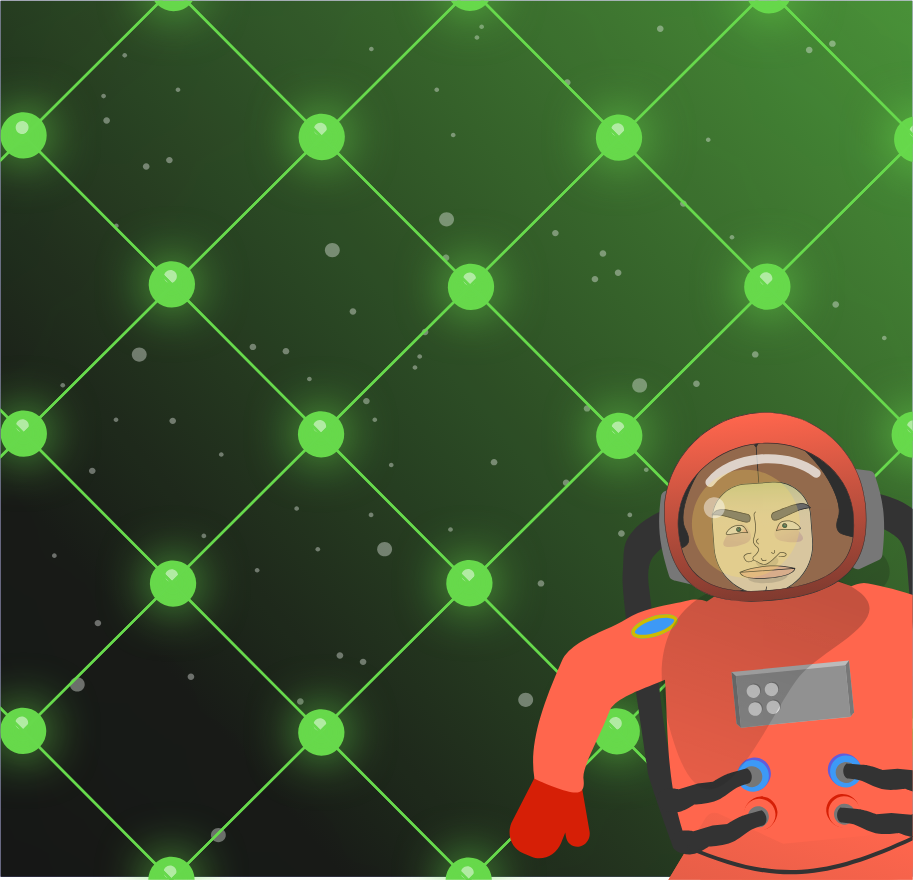As a general strategy, Bev and Alyssa let Crater identify the locations and trajectories of potential targets, while they attempt to stay in the same reference frame and make the shots. Crater also helps Bev and Alyssa keep their timers synchronized if they end up in different reference frames.

Consequently, Crater stays close to the center of the arena to minimize the propagation delay from positions of players on the other team. He's a sitting duck in the arena frame, so Alyssa and Bev need to cover him while they're also after their opponents.

# Relativistic Laser Tag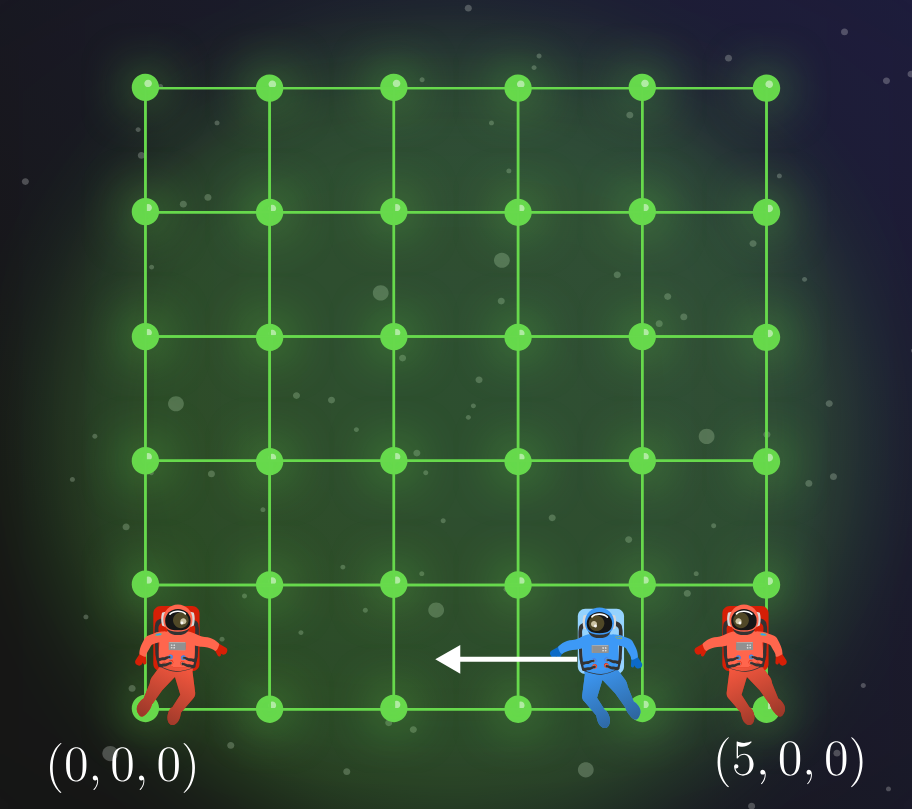The competition commences. Crater identifies the first target, currently moving with speed $\frac c2$ directly toward Alyssa and away from Bev: easy prey.

Crater directs them to both fire as the target crosses $X=\num{3.75}.$ In Alyssa and Bev's reference frame, whose bolt hits the target first?

# Relativistic Laser Tag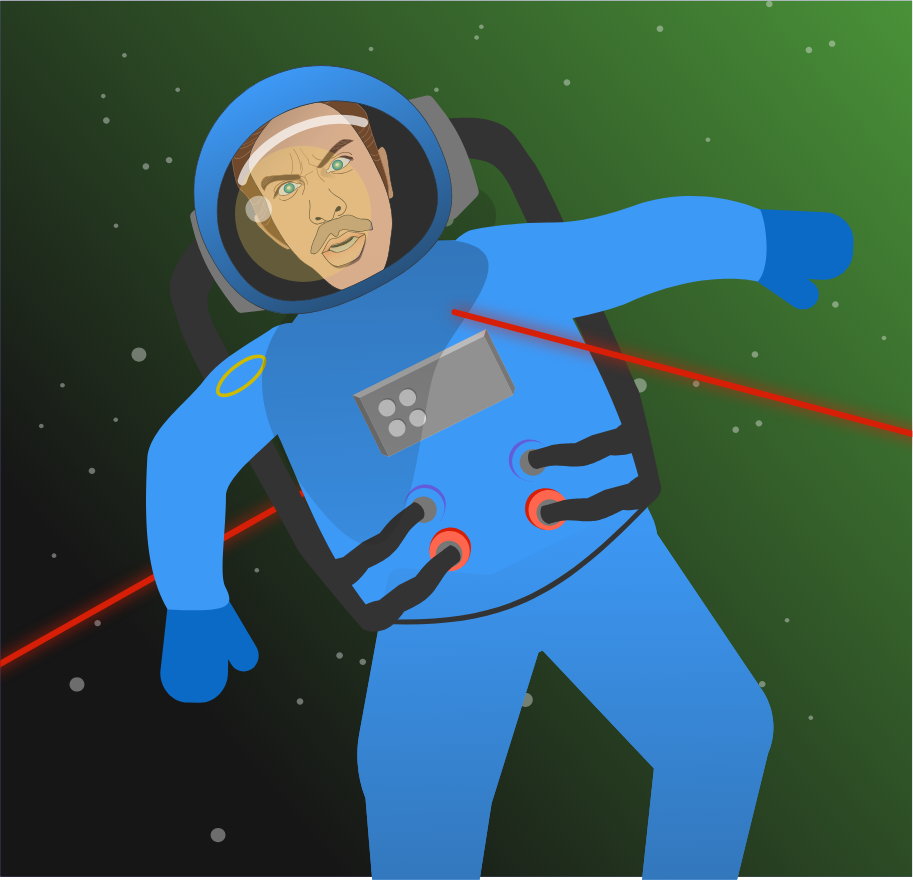Bev says, "Looks like it. I saw our bolts hit him simultaneously, but were they simultaneous in his frame too? I thought we learned at practice that simultaneous events in our reference frame are not simultaneous in others?"

Crater responds, "Yes, that's true, but under certain conditions, two events may be simultaneous in other frames too."

Alyssa retorts, "Well, it would have been helpful to know these conditions before we started playing...."

# Relativistic Laser Tag

We can help Alyssa and Bev unravel the so-called relativity of simultaneity for observers in different frames.They fire their lasers at the same time $T$ from coordinates $X_A=0$ and $X_B=5,$ respectively, but in target's frame, these events are separated in time by $\Delta T^\prime.$ By performing a Lorentz transformation, calculate the time delay in the target's frame (in $\si{\second}$) between when Bev and Alyssa pull their triggers.

Details: The time coordinates of events in the target's frame are found using $T^\prime = \gamma\left( T - \frac{vX}{c^2}\right).$ The target is moving toward Alyssa with speed $V=\frac c2.$

# Relativistic Laser Tag

We just found that if two events A and B are simultaneous in reference frame $F$, then the time difference in another frame $F^\prime$ is $\boxed{\Delta T^\prime = \dfrac{\gamma v \Delta X}{c^2}},$ where $\Delta X = X_B - X_A$ is their separation in $F$, and $v$ is the speed of $F^\prime$ relative to $F.$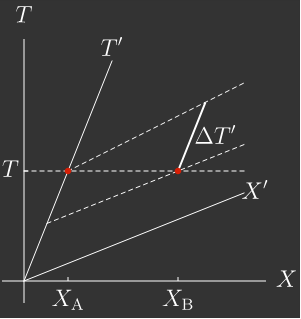According to this result, when an opponent is hit with simultaneous laser bolts in Alyssa and Bev's reference frame, the opponent also detects a (nearly) simultaneous hit in his frame.

Of course, it would be hard for Bev and Alyssa to hit the same point on their opponent's spacesuit, so $\Delta X$ may be a meter or two (roughly, one body-length). However, the $c^2$ in the denominator ensures that the time difference in the opponent's frame would be so small that only the most sensitive equipment could detect it.

Thus, from now on, we will assume that a simultaneous hit in any frame is sufficient to take out an opponent.

# Relativistic Laser Tag

Crater is frantic on the comm link: "Incoming! target at $(5,0,5),$ Bev, heading toward you. Speed $\frac c2$. Another at...."

Now they just hear static on the link. Crater was just ambushed while Bev and Alyssa were distracted by simultaneity; now Bev is in the crosshairs.

"Alyssa, prepare to shoot him at $(5,0,0),$" Bev says as she boosts into a frame heading directly toward her opponent with speed $\frac c2.$ "I'm going to fire back over my shoulder after I pass him."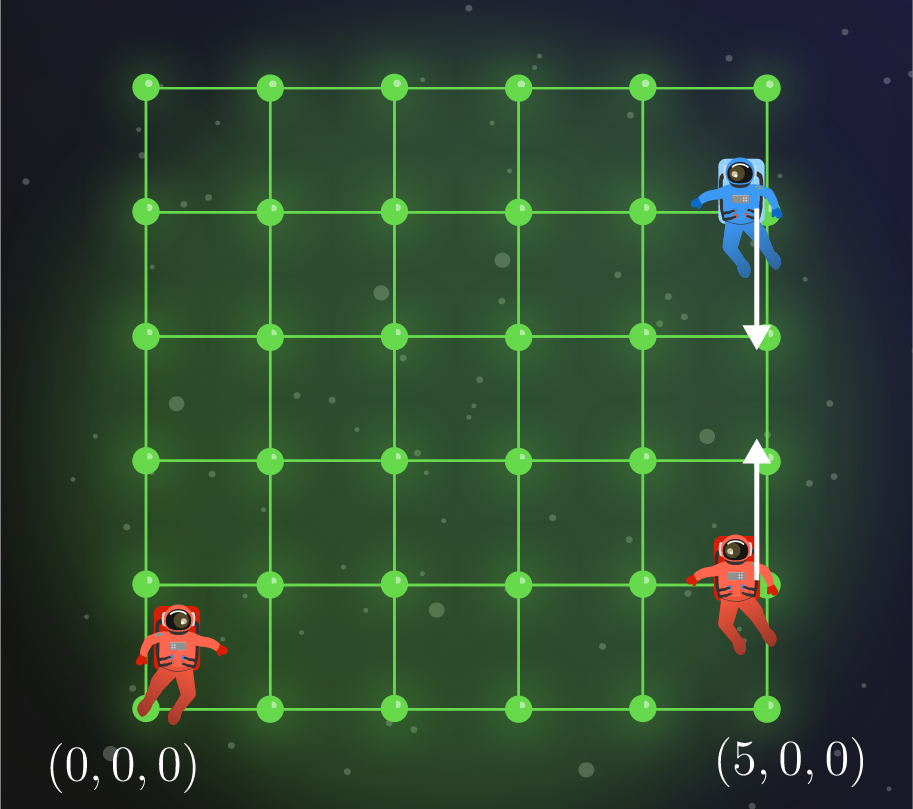Alyssa times her shot toward $(5,0,0)$ to arrive exactly $\SI{10}{\second}$ after Bev's boost. Bev counts off in her frame too. How long after her boost should she shoot over her shoulder to hit her opponent, simultaneous with Alyssa's shot?

# Relativistic Laser Tag

As she awaits confirmation of her shot, Alyssa has a moment of panic.

If Bev is moving away from the target with speed $\frac c2,$ and the target is moving away from Bev with the same speed, then wouldn't the target's speed in Bev's frame be $\frac{c}{2} -\left( - \frac{c}{2} \right) = c?$ This is, of course, what Galilean relativity would predict, and it seems Bev's over-the-shoulder shot would never catch up to her target...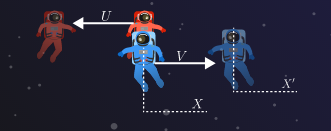Let's help Alyssa calculate the target's velocity in Bev's reference frame. Consider a reference frame with its origin on the point where Bev and the target pass each other. Bev has speed $V=\frac c2$ in this frame, and their opponent has speed $U=\frac c2$ in the opposite direction.

At time $\Delta T$ after they pass, the opponent has moved to a point with coordinates $(\Delta T,-U\Delta T).$ What is the $X^\prime$-coordinate of this point in Bev's frame?

Details: According to the Lorentz transformation, the spatial coordinates of events in Bev's frame are found using $X^\prime = \gamma\left( X - VT\right).$

# Relativistic Laser TagThe target moves to $X^\prime$ after $\Delta T$ passes in Alyssa's frame, but this is not the time Bev would measure. Using the Lorentz transformation of the time coordinate $T^\prime = \gamma\left( \Delta T + \frac{UV\Delta T}{c^2}\right).$

The velocity $U^\prime$ Bev would measure can be formed from $T^\prime$ and $X^\prime :$ $U^\prime = \frac{X^\prime}{T^\prime}.$ How fast is her opponent moving in her reference frame?

Details: Both $U$ and $V$ are $\frac c2.$

# Relativistic Laser Tag

Bev's over-the-shoulder trick shot is timed perfectly, and it catches up to her target just as he arrives at $\left(5,0,0\right),$ simultaneous with Alyssa's shot. The team scores their second KO, and they are victorious in the match!

As they fetch the immobilized Crater, Alyssa and Bev marvel at the array of effects encoded in the Lorentz transformation, and by association, Einstein's postulates. In this quiz, we discovered that simultaneous events in one frame can be highly asynchronous when they are separated by a large distance.

More strikingly, we also saw that under Lorentz transformations relative velocities do not add directly, as in Galilean relativity. In fact, when Bev has velocity $V$ (in Alyssa's frame) and her opponent has velocity $U$ in the opposite direction, Bev measures her opponent's relative velocity as $U^\prime=\frac{U+V}{1+\dfrac{UV}{c^2}}.$ This is called the addition rule for relativistic velocities, and we will revisit it in a later quiz.

# Relativistic Laser Tag

×